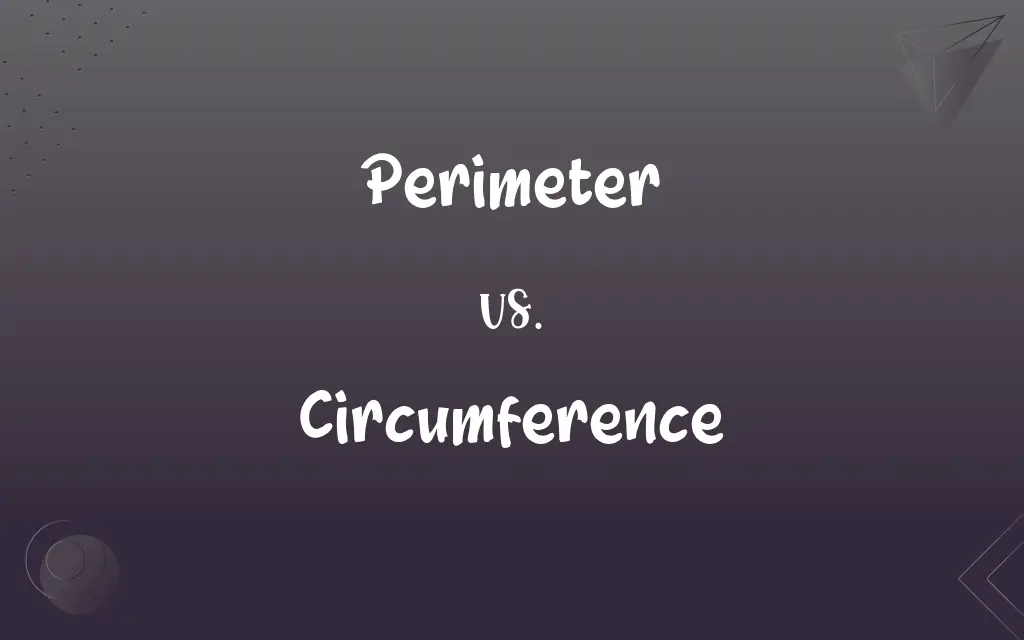# Perimeter vs. Circumference: What's the Difference?Edited by Sawaira Riaz || By Sumera Saeed || Updated on November 8, 2023
The perimeter is the total distance around a two-dimensional shape, while circumference specifically refers to the distance around a circle.## Key Differences

The perimeter is a term used to describe the total distance around any two-dimensional geometric shape, such as a rectangle, square, or triangle. Circumference, however, is a term that applies specifically to the distance around a circle or circular object. It is the equivalent of the perimeter but for round shapes.
In practical applications, one might measure the perimeter of a garden to determine how much fencing is needed. On the other hand, one might measure the circumference of a pipe to ensure a proper fit for materials that need to wrap around it, such as insulation or decals.
The concept of perimeter can be extended to include irregular shapes and even non-geometric areas, such as the boundary of a property. Circumference remains strictly within the realm of circles and does not apply to shapes that do not have a constant distance from a central point.
In essence, the perimeter is a more general concept used in various branches of mathematics and everyday measurements, whereas circumference is a specific type of perimeter used exclusively for circles and circular objects.

## Comparison Chart

### Definition

The total length around a two-dimensional shape
The distance around a circle

### Applicability

Any two-dimensional shape
Only circular shapes

### Geometric Use

Used for polygons and irregular shapes
Used exclusively for circles

### Real-world Example

Measuring fencing for a rectangular yard
Measuring the edge of a circular pool cover

## Perimeter and Circumference Definitions

#### Perimeter

The outermost limits or boundary of an area or object.
The soldiers guarded the perimeter of the camp.

#### Circumference

The distance around the edge of a circle.
The circumference of the circular garden was 30 feet.

#### Perimeter

A fortified boundary that protects or defines an area.
The perimeter was secured to prevent any breaches.

#### Circumference

The outer boundary, especially of a circular area.
The circumference of the base of the cylinder was measured.

#### Perimeter

The continuous line forming the boundary of a closed geometric figure.
They calculated the perimeter of the square field.

#### Circumference

The measurement around the middle of something, especially a body part.
The tailor took the circumference of her waist for the dress.

#### Perimeter

The boundary of a specific area or surface.
The dogs were allowed to roam within the perimeter of the backyard.

#### Circumference

The length of such a boundary.
The wheel's circumference was exactly two meters.

#### Perimeter

The sum of the lengths of the sides of a polygon.
For her math homework, she found the perimeter of various polygons.

#### Circumference

A line enclosing a circular space.
The children drew the circumference of the circle with a compass.

#### Perimeter

A closed line bounding a plane area.

#### Circumference

The boundary line of a circle.

#### Perimeter

The length of such a line.

#### Circumference

The boundary line of an area or object.

#### Perimeter

The outer limits of an area.

#### Perimeter

A defended boundary protecting a military position.

## FAQs

#### Can the terms perimeter and circumference be used interchangeably?

No, perimeter refers to any shape, while circumference is specific to circles.

#### Is the circumference always larger than the perimeter?

Not necessarily; it depends on the size of the respective shapes.

#### How do you measure the perimeter of a rectangle?

Add the lengths of all four sides of the rectangle.

#### Why do we need to calculate the circumference?

Calculating circumference is important for things like fitting circular objects together.

#### Does the complexity of a shape affect how you measure its perimeter?

Yes, more complex shapes may require more detailed measurements.

#### Can you find the perimeter of a circle?

The term perimeter is not typically used for circles; circumference is the correct term.

#### Is the circumference a type of perimeter?

Yes, it is the perimeter of a circle.

#### Are perimeter and circumference both considered linear measurements?

Yes, both are linear measurements despite the shape they are measuring.

#### When do you need to know the perimeter?

When you need the measure of the boundary of any flat, two-dimensional shape.

#### Can the formula for circumference be applied to an ellipse?

No, an ellipse has a different formula for calculating the distance around it.

#### Can circumference be measured in three dimensions?

No, circumference is also a two-dimensional measurement, despite the circle being represented in three dimensions.

#### When do you need to know the circumference?

When dealing with the distance around a circle or circular object.

#### Can both perimeter and circumference be measured in the same units?

Yes, both are measured in linear units, such as meters or feet.

#### Can you have a shape with an infinite perimeter but finite circumference?

No, shapes with a finite circumference will also have a finite perimeter.

#### Are perimeters always flat measurements?

Yes, perimeter is a two-dimensional measurement.

#### What's a common mistake when measuring perimeter or circumference?

A common mistake is using the wrong formula or not measuring accurately.

#### Does the complexity of a shape affect its circumference?

Circumference is only used for circles and is always measured the same way.